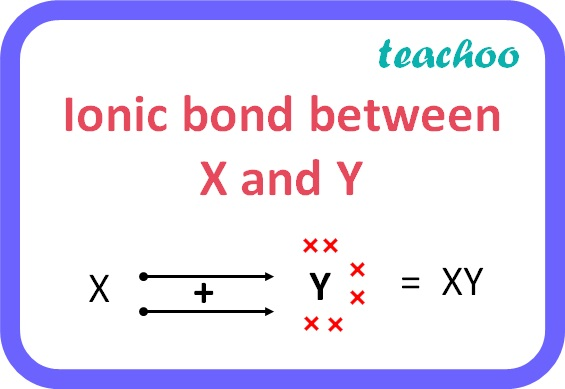Past Year - 3 Mark Questions

Class 10
Chapter 5 Class 10 - Periodic Classification of Elements (Term 2)

## Two elements X and Y have atomic numbers 12 and 16 respectively. To which period of the modern periodic table do these two elements belong? What type of bond will be formed between them and why? Also give the chemical formula of the compound formed.

Atomic number of X = 12

Electronic configuration = 2, 8, 2

Atomic number of Y = 16

Electronic configuration = 2, 8, 6

Since both X and Y have 3 shells, they both belong to the third period.

In order to attain noble gas configuration, X will lose 2 electrons whereas Y will gain 2 electrons . Therefore, X and Y will form an ionic bond which each other i.e. Y will accept the two electrons donated by X.The chemical formula of the compound formed is XY